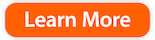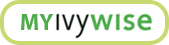Here’s What Students Need to Know About the ACT Math Section

By IvyWise Master Tutor

If you’re preparing to take the ACT this fall, it’s important to understand the exam’s content, format, and test-taking strategies in order to maximize your performance on test day. The ACT Math section is a great entry point for students in order to understand how the ACT is organized as well as the test’s key differences from the SAT.

At IvyWise we encourage students who are preparing for standardized testing to take a diagnostic exam of both that ACT and SAT in order to determine which test is the best-fit for students’ abilities. Before taking a diagnostic, however, it’s important to understand both the SAT and ACT’s content and structure – especially for subjects, like math, that can intimidate many students.

Here’s a breakdown of the ACT Math section, and some additional information on how it compares to the SAT Math section.

The Basics

The ACT Math section is very straightforward – 60 minutes with 60 questions ranging from pre-algebra and algebra to geometry and basic trigonometry. Formulas are not provided, and word problems and geometry questions are given particular emphasis in the ACT Math section. Students may use a calculator on the test, even though all of the questions are designed to be solved without one (certain advanced calculators such as the TI-89 are not permitted.)

The ACT Math section presents multiple-choice questions that require students to use reasoning skills to solve practical problems in math. Students need knowledge of basic formulas (such as the distance formula) and computational skills to answer the problems. However, students are not required to know complex formulas (such as the quadratic equation) or to perform extensive arithmetic work.

Timing and Questions

The ACT Math section is, like other ACT sections, designed so that most students will not have enough time to answer all the questions. Unlike any other ACT section, the ACT Math section is tiered by levels of difficulty – questions 1-20 are designed to be the least time consuming, questions 21-40 are moderately time consuming, and questions 41-60 are the most time consuming. The section is designed to assess the math skills that a student would typically acquire up to grade 12, though the test’s primary difficulty often lies in the unfamiliar way that the questions are worded. That means that though a question might be testing basic concepts such as exponents, ratios, averages, or percentages, the way in which these questions are phrased, or the word problems in which they are embedded, will often times be challenging to decipher.

ACT Math vs. SAT Math

What is the main difference between ACT and SAT Math sections? Perhaps the biggest difference is that the ACT Math section places more of an emphasis on word problems and geometry, whereas the SAT Math section places more emphasis on algebra. In terms of structure, the SAT Math section is divided into two separate sections – one with calculator and one without. The SAT Math section also includes non-multiple-choice sections in which students write in their answer.

The other key main difference is time – the SAT does provide more time per question – though the questions can often times be more time consuming.

 ACT Math SAT Math Number of questions 60 58 (total) Time per question 1 minute 1 minute 23 seconds Algebra Content (based on official released tests) 45% 60%

Take note of these important features and differences when beginning to prepare for the ACT, or when deciding between the ACT and SAT. While no feature of either math section is decidedly more or less ‘easy,’ the ACT Math section does have some pros – and cons – for many students, and that could influence students’ decision whether or not to take the ACT at all.

Are you prepared for your standardized testing? Get on track with your test prep with our free Tutoring Checklist. Fill out the form below!